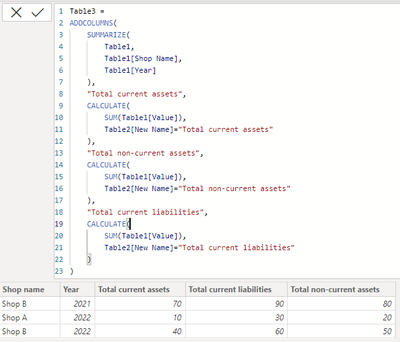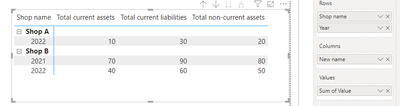cancel
Showing results for
Did you mean:

Fabric is Generally Available. Browse Fabric Presentations. Work towards your Fabric certification with the Cloud Skills Challenge.Frequent Visitor

## New table using values from two other tables

Hello,

I am working on analysing businesses' financial statements and need some help with writing DAX code to make a new table using values from another table, and names from another table. I already posted a similar question (New column in new table based on values of other tables ) but my problem has gotten a bit more complex so the solution there doesn't apply to this. Maybe @Mahesh0016 has an answer for this as well? 😄

Say I have two tables, Table 1 and Table 2.

Table 1 (which has info on shop names, year, the original line item name from each shop's financial statements and the value corresponding to that line item)

 Shop name Year Line item name Value Shop A 2022 current assets total 10 Shop A 2022 non-current assets total 20 Shop A 2022 current liabilities 30 Shop B 2022 CA total 40 Shop B 2022 NCA total 50 Shop B 2022 CL total 60 Shop B 2021 CA total 70 Shop B 2021 NCA total 80 Shop B 2021 CL total 90

Table 2 (which has the original line item name and a new name for consistency)

 Line item name New name current assets total Total current assets CA total Total current assets non-current assets total Total non-current assets NCA total Total non-current assets current liabilities total Total current liabilities CL total Total current liabilities

I want to use information from Tables 1 and 2 to ultimately get a table like this (let's call it Table 3):

Table 3

 Shop name Year Total current assets Total non-current liabilities Total current liabilities Shop A 2022 10 20 30 Shop B 2022 40 50 60 Shop B 2021 70 80 90

1 ACCEPTED SOLUTIONSuper User

hi @a22

something like this?

``````Table3 =
SUMMARIZE(
Table1,
Table1[Shop Name],
Table1[Year]
),
"Total current assets",
CALCULATE(
SUM(Table1[Value]),
Table2[New Name]="Total current assets"
),
"Total non-current assets",
CALCULATE(
SUM(Table1[Value]),
Table2[New Name]="Total non-current assets"
),
"Total current liabilities",
CALCULATE(
SUM(Table1[Value]),
Table2[New Name]="Total current liabilities"
)
)``````4 REPLIES 4Super User

hi @a22

try to plot a matrix visual directly, like:or?Frequent Visitor

Thanks, that's a good idea! But I would prefer to do it without having to plot a visual....is there a way to just use DAX to make the table?

Reason is I need this table to add to my data model. If I were to plot a matrix visual I'd have to save it as a csv and then load that into my model which I'd rather avoid doing.Super User

hi @a22

something like this?

``````Table3 =
SUMMARIZE(
Table1,
Table1[Shop Name],
Table1[Year]
),
"Total current assets",
CALCULATE(
SUM(Table1[Value]),
Table2[New Name]="Total current assets"
),
"Total non-current assets",
CALCULATE(
SUM(Table1[Value]),
Table2[New Name]="Total non-current assets"
),
"Total current liabilities",
CALCULATE(
SUM(Table1[Value]),
Table2[New Name]="Total current liabilities"
)
)``````Frequent Visitor

Thanks so much!Announcements#### Power BI Monthly Update - November 2023

Check out the November 2023 Power BI update to learn about new features.#### Fabric Community News unified experience

Read the latest Fabric Community announcements, including updates on Power BI, Synapse, Data Factory and Data Activator.#### The largest Power BI and Fabric virtual conference

130+ sessions, 130+ speakers, Product managers, MVPs, and experts. All about Power BI and Fabric. Attend online or watch the recordings.Top Solution Authors
Top Kudoed Authors
Users online (3,697)